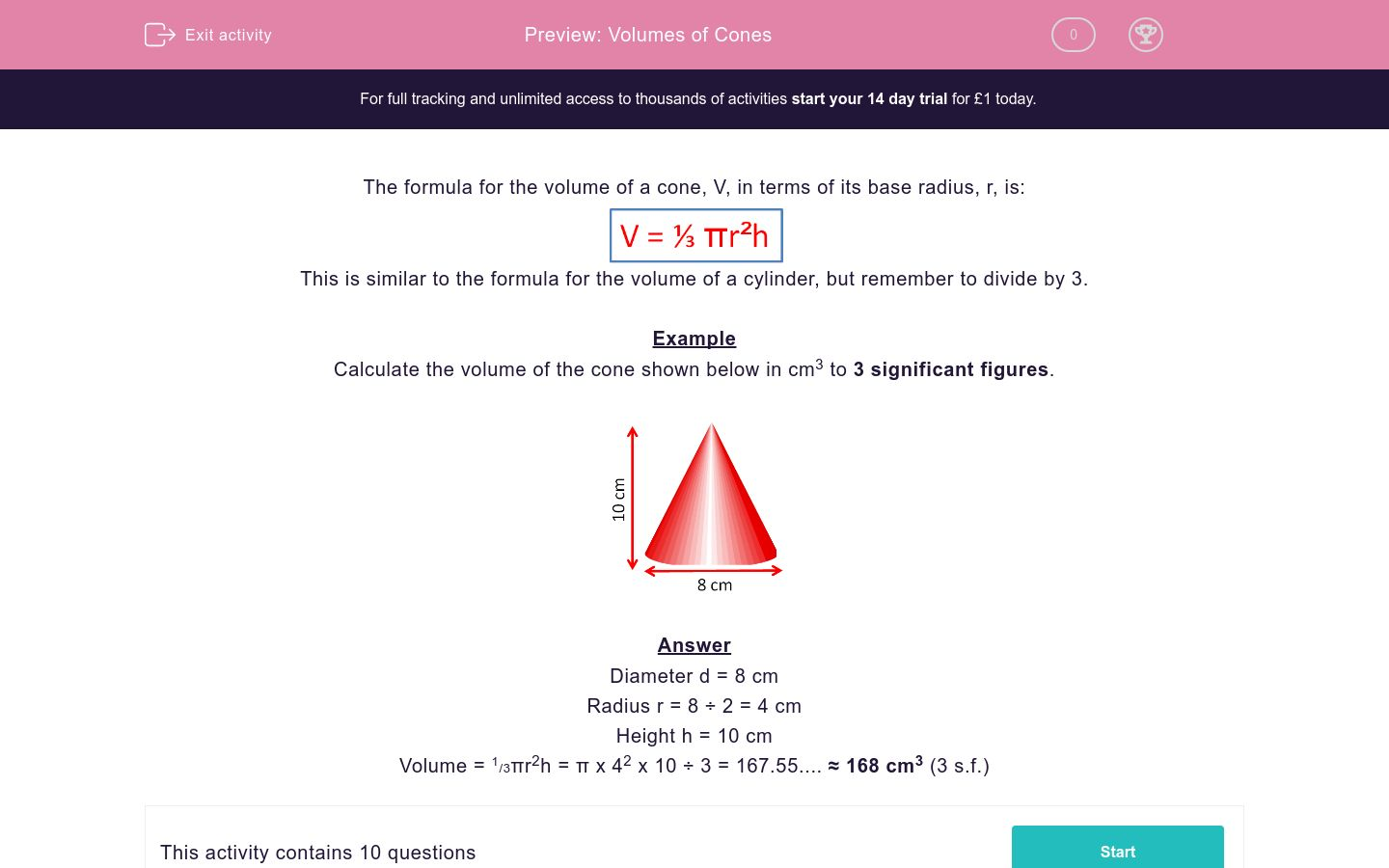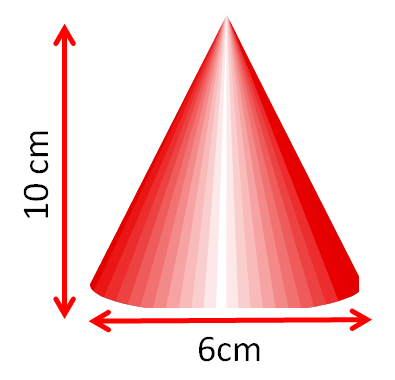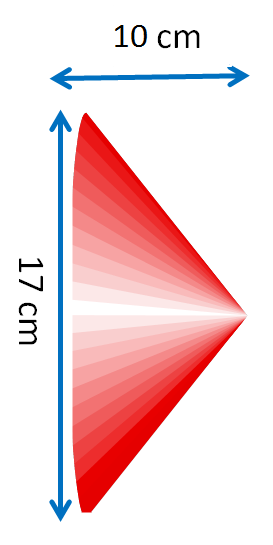# Volumes of Cones

In this worksheet, students calculate the volume of cones, given their height and base diameter.Key stage:  KS 2

Curriculum topic:   Maths and Numerical Reasoning

Curriculum subtopic:   Volume and Capacity

Difficulty level:### QUESTION 1 of 10

The formula for the volume of a cone, V, in terms of its base radius, r, is:This is similar to the formula for the volume of a cylinder, but remember to divide by 3.

Example

Calculate the volume of the cone shown below in cm3 to 3 significant figures.Diameter d = 8 cm

Radius r = 8 ÷ 2 = 4 cm

Height h = 10 cm

Volume = 1/3πr2h = π x 42 x 10 ÷ 3 = 167.55.... ≈ 168 cm3 (3 s.f.)

Calculate the volume of the cone shown below in cm3 to 3 sig. figs.

Just write the number.Calculate the volume of the cone shown below in cm3 to 3 sig. figs.

Just write the number.Calculate the volume of the cone shown below in cm3 to 3 sig. figs.

Just write the number.Calculate the volume of the cone shown below in cm3 to 3 sig. figs.

Just write the number.Calculate the volume of the cone shown below in cm3 to 3 sig. figs.

Just write the number.Calculate the volume of the cone shown below in cm3 to 3 sig. figs.

Just write the number.Calculate the volume of the cone shown below in cm3 to 3 sig. figs.

Just write the number.Calculate the volume of the cone shown below in cm3 to 3 sig. figs.

Just write the number.Calculate the volume of the cone shown below in cm3 to 3 sig. figs.

Just write the number.Calculate the volume of the cone shown below in cm3 to 3 sig. figs.

Just write the number.• Question 1

Calculate the volume of the cone shown below in cm3 to 3 sig. figs.

Just write the number.94.2
EDDIE SAYS
Vol = π × 3² × 10 ÷ 3
• Question 2

Calculate the volume of the cone shown below in cm3 to 3 sig. figs.

Just write the number.104
EDDIE SAYS
Vol = π × 3² × 11 ÷ 3
• Question 3

Calculate the volume of the cone shown below in cm3 to 3 sig. figs.

Just write the number.72.0
EDDIE SAYS
Vol = π × 2.5² × 11 ÷ 3
• Question 4

Calculate the volume of the cone shown below in cm3 to 3 sig. figs.

Just write the number.605
EDDIE SAYS
Vol = π × 8.5² × 8 ÷ 3
• Question 5

Calculate the volume of the cone shown below in cm3 to 3 sig. figs.

Just write the number.85.1
EDDIE SAYS
Vol = π × 2.5² × 13 ÷ 3
• Question 6

Calculate the volume of the cone shown below in cm3 to 3 sig. figs.

Just write the number.128
EDDIE SAYS
Vol = π × 3.5² × 10 ÷ 3
• Question 7

Calculate the volume of the cone shown below in cm3 to 3 sig. figs.

Just write the number.37.7
EDDIE SAYS
Vol = π × 2² × 9 ÷ 3
• Question 8

Calculate the volume of the cone shown below in cm3 to 3 sig. figs.

Just write the number.18.8
EDDIE SAYS
Vol = π × 1.5² × 8 ÷ 3
• Question 9

Calculate the volume of the cone shown below in cm3 to 3 sig. figs.

Just write the number.757
EDDIE SAYS
Vol = π × 8.5² × 10 ÷ 3
• Question 10

Calculate the volume of the cone shown below in cm3 to 3 sig. figs.

Just write the number.205000
EDDIE SAYS
Vol = π × 62.5² × 50 ÷ 3
---- OR ----